Test: Queues & Stacks

# Test: Queues & Stacks - Computer Science Engineering (CSE)

Test Description

## 25 Questions MCQ Test GATE Computer Science Engineering(CSE) 2024 Mock Test Series - Test: Queues & Stacks

Test: Queues & Stacks for Computer Science Engineering (CSE) 2023 is part of GATE Computer Science Engineering(CSE) 2024 Mock Test Series preparation. The Test: Queues & Stacks questions and answers have been prepared according to the Computer Science Engineering (CSE) exam syllabus.The Test: Queues & Stacks MCQs are made for Computer Science Engineering (CSE) 2023 Exam. Find important definitions, questions, notes, meanings, examples, exercises, MCQs and online tests for Test: Queues & Stacks below.
Solutions of Test: Queues & Stacks questions in English are available as part of our GATE Computer Science Engineering(CSE) 2024 Mock Test Series for Computer Science Engineering (CSE) & Test: Queues & Stacks solutions in Hindi for GATE Computer Science Engineering(CSE) 2024 Mock Test Series course. Download more important topics, notes, lectures and mock test series for Computer Science Engineering (CSE) Exam by signing up for free. Attempt Test: Queues & Stacks | 25 questions in 75 minutes | Mock test for Computer Science Engineering (CSE) preparation | Free important questions MCQ to study GATE Computer Science Engineering(CSE) 2024 Mock Test Series for Computer Science Engineering (CSE) Exam | Download free PDF with solutions
 1 Crore+ students have signed up on EduRev. Have you?
Test: Queues & Stacks - Question 1

### A queue is implemented using an array such that ENQUEUE and DEQUEUE operations are performed efficiently. Which one of the following statements is CORRECT ( n refers to the number of items in the queue)?

Detailed Solution for Test: Queues & Stacks - Question 1

Answer A - Circular Queue Implementation

Both operations can be performed in O(1) time in Circular Queue implimentation where Enqueue and Dequeue operation are done at last node. Single pointer needed at last node.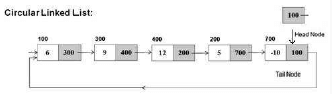Test: Queues & Stacks - Question 2

### Consider the following statements: i. First-in-first-out types of computations are efficiently supported by STACKS. ii. Implementing LISTS on linked lists is more efficient than implementing LISTS on an array for almost all the basic LIST operations. iii. Implementing QUEUES on a circular array is more efficient than implementing QUEUES on a linear array with two indices. iv. Last-in-first-out type of computations are efficiently supported by QUEUES.

Test: Queues & Stacks - Question 3

### What is the minimum number of stacks of size  required to implement a queue of size ?

Detailed Solution for Test: Queues & Stacks - Question 3

A queue can be implemented using two stacks.
Let queue be represented as " q "
and stacks used to implement q be "stack1" and "stack2".
q can be implemented in two ways:
Method 1 (By making EnQueue operation costly)

This method makes sure that newly entered element is always at the bottom of stack 1, so that deQueue operation just pops from stack1. To put the element at top of stack1, stack2 is used.

enQueue(q, x)

1) While stack1 is not empty, push everything from satck1 to stack2.
2) Push x to stack1 (assuming size of stacks is unlimited).
3) Push everything back to stack1.

dnQueue(q)

1) If stack1 is empty then error
2) Pop an item from stack1 and return it

Method 2 (By making deQueue operation costly)

In this method, in en-queue operation, the new element is entered at the top of stack1. In de-queue operation, if stack2 is
empty then all the elements are moved to stack2 and finally top of stack2 is returned.

enQueue(q, x)

1) Push x to stack1 (assuming size of stacks is unlimited).

deQueue(q)

1) If both stacks are empty then error.
2) If stack2 is empty
While stack1 is not empty, push everything from satck1 to stack2.
3) Pop the element from stack2 and return it.

Test: Queues & Stacks - Question 4

An implementation of a queue Q, using two stacks S1 and S2, is given below:

void insert (Q, x) {

push (S1, x);

}

void delete (Q) {

if (stack-empty(S2)) then

if (stack-empty(S1)) then {

print(“Q is empty”);

return;

}

else while (!(stack-empty(S1))){

x=pop(S1);

push(S2,x);

}
x=pop(S2);

}

let n insert and  m(≤  n)delete operations be performed in an arbitrary order on an empty queue Q. Let x and  y be the number of push and pop operations  performed respectively in the process. Which one of the following is true for all m and n?

Detailed Solution for Test: Queues & Stacks - Question 4

The order in which insert and delete operations are performed matters here.
The best case: Insert and delete operations are performed alternatively. In every delete operation, 2 pop and 1 push
operations are performed. So, total m+ n push (n push for insert() and m push for delete()) operations and 2m pop operations are performed.
The worst case: First n elements are inserted and then m elements are deleted. In first delete operation, n + 1 pop operations and n push operation are performed. Other than first, in all delete operations, 1 pop operation is performed. So, total m + n pop operations and 2n push operations are performed (n push for insert() and m push for delete())

Test: Queues & Stacks - Question 5

Suppose you are given an implementation of a queue of integers. The operations that can be performed on the queue are:

i. isEmpty (Q) — returns true if the queue is empty, false otherwise.

ii. delete (Q) — deletes the element at the front of the queue and returns its value.

iii. insert (Q, i) — inserts the integer i at the rear of the queue.

Consider the following function:

void f (queue Q) {

int i ;

if (!isEmpty(Q)) {

i = delete(Q);

f(Q);

insert(Q, i);

}
}

What operation is performed by the above function f ?

Detailed Solution for Test: Queues & Stacks - Question 5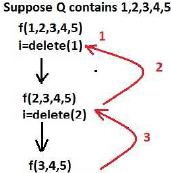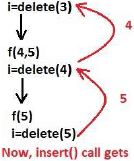insert() will inserts the value in just reverse order.

Test: Queues & Stacks - Question 6

Suppose a circular queue of capacity (n - 1) elements is implemented with an array of n elements. Assume that the insertion and deletion operations are carried out using REAR and FRONT as array index variables, respectively. Initially, REAR = FRONT = 0. The conditions to detect queue full and queue empty are

Detailed Solution for Test: Queues & Stacks - Question 6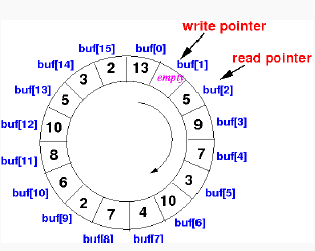rear = Write
full: (REAR+1) mod n == FRONT
empty: REAR == FRONT
Only option A matches.

Test: Queues & Stacks - Question 7

Consider the following operation along with Enqueue and Dequeue operations on queues, where k is a global parameter.

MultiDequeue(Q){

m = k

while (Q is not empty) and (m > 0) {

Dequeue(Q)

m = m – 1

}

}

What is the worst case time complexity of a sequence of n queue operations on an initially empty queue?

Detailed Solution for Test: Queues & Stacks - Question 7

There are three possible operations on queue- Enqueue, Dequeue and MultiDequeue. MultiDequeue is calling Dequeue multiple times based on a global variable k. Since, the queue is initially empty, whatever be the order of these operations, there cannot be more no. of Dequeue operations than Enqueue operations. Hence, the total no. operations will be  n only.

Test: Queues & Stacks - Question 8

A circular queue has been implemented using a singly linked list where each node consists of a value and a single pointer pointing to the next node. We maintain exactly two external pointers FRONT and REAR pointing to the front node and the rear node of the queue, respectively. Which of the following statements is/are CORRECT for such a circular queue, so that insertion and deletion operations can be performed in O(1) time?

(I) Next pointer of front node points to the rear node.

(II) Next pointer of rear node points to the front node.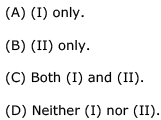Detailed Solution for Test: Queues & Stacks - Question 8

Answer is Next pointer to Rear node has Pointer to Front node.
Hence, only  II is correct.

Test: Queues & Stacks - Question 9

Choose the correct alternatives (more than one may be correct) and write the corresponding letters only:

The following sequence of operations is performed on a stack:

PUSH  (10), PUSH (20), POP, PUSH (10), PUSH (20), POP, POP, POP, PUSH (20), POP

The sequence of values popped out is

Detailed Solution for Test: Queues & Stacks - Question 9

Lets try something different when you read the word pop then delete the last pushed element and print it ..now delete the push word which we have already executed ..now go on from left to right and do the same
So, Output will be 20, 20, 10, 10, 20

Test: Queues & Stacks - Question 10

Which of the following permutations can be obtained in the output (in the same order) using a stack assuming that the input is the sequence  1,2,3,4,5 in that order?

Detailed Solution for Test: Queues & Stacks - Question 10

push 1 push 2 push 3 pop 3 push 4 pop 4 push 5 pop 5 pop 2 pop 1 then o/p is 3,4,5,2,1 option b

Test: Queues & Stacks - Question 11

The postfix expression for the infix expression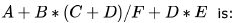Detailed Solution for Test: Queues & Stacks - Question 11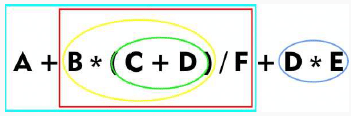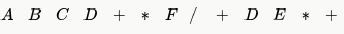Test: Queues & Stacks - Question 12

A priority queue Q is used to implement a stack that stores characters. PUSH (C) is implemented as INSERT (Q, C, K) where K is an appropriate integer key chosen by the implementation. POP is implemented as DELETEMIN(Q). For a sequence of operations, the keys chosen are in

Detailed Solution for Test: Queues & Stacks - Question 12

Implementing stack using priority queue require first element inserted in stack will be deleted at last, and to implement it using deletemin() operation of queue will require first element inserted in queue must have highest priority.
So the keys must be in strictly decreasing order.

Test: Queues & Stacks - Question 13

Let S be a stack of size n ≥1. Starting with the empty stack, suppose we push the first n natural numbers in sequence, and then perform n pop operations. Assume that Push and Pop operations take X seconds each, and Y seconds elapse between the end of one such stack operation and the start of the next operation. For m ≥1, define the stack-life of m as the time elapsed from the end of Push(m) to the start of the pop operation that removes m from S. The average stack-life of an element of this stack is

Detailed Solution for Test: Queues & Stacks - Question 13

let us represent stack-life of ith element as S(i). The ith element will be in stack till (n-i) elements are pushed and popped. Plus one more Y for the time interval between the push of   ith  element and the i+1th element. So,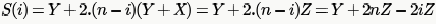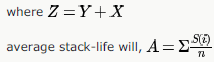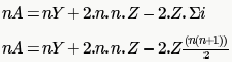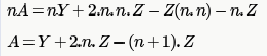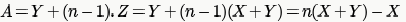Test: Queues & Stacks - Question 14

A single array A[1 .. MAXSIZE] is used to implement two stacks. The two stacks grow from opposite ends of the array.
Variables top1 and top 2 (top < top 2) point to the location of the topmost element in each of the stacks. If the space is to be used efficiently, the condition for “stack full” is

Detailed Solution for Test: Queues & Stacks - Question 14

Since the stacks are growing from opposite ends, initially top1 = 1 and top2 = MAXSIZE. Now, to make the space usage most efficient we should allow one stack to use the maximum possible space as long as other stack doesn't need it. So, either of the stack can grow as long as there is space on the array and hence the condition must be top1 = top2 - 1;

Test: Queues & Stacks - Question 15

Assume that the operators +, , X  are left associative and  is right associative. The order of precedence (from highest to lowest) is , X, +, -. The postfix expression corresponding to the infix expression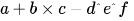is

Detailed Solution for Test: Queues & Stacks - Question 15

Here is the procedure first :
Scan Infix Expression from left to right whenever you see operand just print it.
But In case of operator
if(stack is empty) then push it.
if(precedence(tos) < precedence(current operator) ) push it.
else if (precedence(tos) > precedence(current operator) ) pop and print.

else if (precedence(tos) == precedence(current operator) ) then check for associativity.In case Operators are Left to right
then pop and print it otherwise push the current operator (Right to Left Associativity)
And once you have scanned infix expression completely. Make sure pop all the element and print it in same order.
Here the infix expression is a+b×c−d^e^f
a : print it
+ : push into the Operator Stack
b : print it
* : its having higher precedence than + then push into Operator Stack
c : print it
'-' : '-' is having less precedence than '*' so pop from operator stack and print '*'.after this stack will be having '+' on
top.which is having same precedence as '-' but both are left to right associative then just pop + and print it.Now stack is
empty. Push '-' to it.
d : print it
'^' : top of the stack is having '-' which has lower precedence than '^' so simply push '^' into stack
e : print it.
'^' : Now top of the stack is '^' and has same precedence so associativity will come to picture. Since '^' is right associative
as given in question. So '^' will be pushed.
f : print it.
Now we have scanned entire infix expression.Now pop the stack untill it becomes empty.This way you will get
abc*+def^^-

Test: Queues & Stacks - Question 16

The best data structure to check whether an arithmetic expression has balanced parentheses is a

Detailed Solution for Test: Queues & Stacks - Question 16

STACK Scan the expression from left to right whenever a left paranthesis is encountered just PUSH it into stack and whenever a right paranthesis is encountered just POP it from stack ..if at the end of expression we are left with an empty stack then it is a correctly parenthesized expression

Test: Queues & Stacks - Question 17

A program attempts to generate as many permutations as possible of the string, 'abcd' by pushing the characters  in the same order onto a stack, but it may pop off the top character at any time. Which one of the following strings CANNOT be generated using this program?

Detailed Solution for Test: Queues & Stacks - Question 17

A. push a & pop a, push b & pop b, push c & pop c, and finally push d and pop d
sequence of popped elements will come to abcd
B. first push abcd, and after that pop one by one sequence of popped elements will come to dcba
C. push abc, and after that pop one by one sequence of popped elements will come to cba, now push d and pop d, final
D. this sequence is not possible because 'a' can not be pooped before 'b' any how

Test: Queues & Stacks - Question 18

A function f defined on stacks of integers satisfies the following properties. f(∅) = 0 and f (push (S, i)) = max (f(S), 0) + i for all stacks S and integers i.

If a stack S contains the integers 2, -3, 2, -1, 2 in order from bottom to top, what is f(S)?

Detailed Solution for Test: Queues & Stacks - Question 18

i Element to be pushed

Initial State f(φ) = 0 For Empty Stack F(S) is 0.

Then we push each element ( )one by one and calculate f(s)for each insertion as given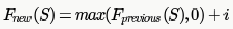Is the function to compute F(s) for each insertions

1. INSERT 2 into Stack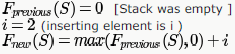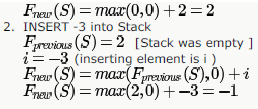Similarly ,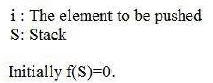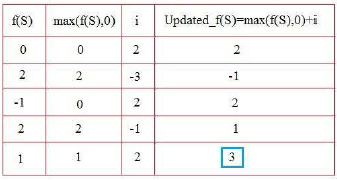The value of F(s) after inserting all elements into stack is 3

Test: Queues & Stacks - Question 19

The following postfix expression with single digit operands is evaluated using a stack:

8 2 3 ^ / 2 3 * + 5 1 * -

Note that ^ is the exponentiation operator. The top two elements of the stack after the first * is evaluated are

Detailed Solution for Test: Queues & Stacks - Question 19

push 8 so stack is 8
push 2 so stack is 8 2
push 8 2 3
^ pop 3 and 2 perform opn 2^3 and push to stack. stack is 8 8
/ pop 8 and 8 perform 8/8 and push result to stack . stack is 1
push 2 stack is 1 2
push 3 stack is 1 2 3
* pop 3 and 2 perform by 2*3 and push . stack is 1 6

Test: Queues & Stacks - Question 20

Consider the following C program:

#include <stdio.h>

#define EOF -1

void push (int); /* push the argument on the stack */

int pop  (void); /* pop the top of the stack */

void flagError ();

int main ()

{                         int c, m, n, r;

while ((c = getchar ()) != EOF)

{ if (isdigit (c) )

push (c);

else if ((c == '+') || (c == '*'))

{          m = pop ();

n = pop ();

r = (c == '+') ? n + m : n*m;

push (r);

}
else if (c != ' ')

flagError ();

}
printf("% c", pop ());

}

What is the output of the program for the following input?

5 2 * 3 3 2 + * +

Detailed Solution for Test: Queues & Stacks - Question 20

B) 25
let first part
5 ----push
2------push
push------5*2=10. (pops 5 and 2)

push 3
push 3
push 2
push 3+2 = 5 (pops 2 and 3)
push 5*3 = 15 (pops (5 and 3)
push 15 + 10 = 25 (pops (15 and 10)

Test: Queues & Stacks - Question 21

Suppose a stack implementation supports an instruction REVERSE, which reverses the order of elements on the stack, in addition to the PUSH and POP instructions. Which one of the following statements is TRUE (with respect to this modified stack)?

Detailed Solution for Test: Queues & Stacks - Question 21

(C) is the answer. While ENQUEUE we REVERSE the stack, PUSH the element and then again REVERSE the stack. For DEQUE we simply POP the element.
(Option (B) can be used to get the first element from the stack by doing a POP after REVERSE for DEQUEUE and PUSH for\ ENQUEUE. But we have to restore the stack using REVERSE (otherwise next POP won't work) which means DEQUEUE actually needs 3 instructions and not 2)

Test: Queues & Stacks - Question 22

The result evaluating the postfix expression 10 5 + 60 6 / * 8 - is

Detailed Solution for Test: Queues & Stacks - Question 22

we have to keep symbol into stack and when we get two operands followed by operator ..we will apply operator on last two operands

symbol                     stack

10                       10 (keep in stack)
5                 10 5 (keep in stack)

+                  10 5 + = 10+5 = 15 ( apply operator on last 2 operands)

60                 15 60 (keep in stack)

6                  15 60 6 (keep in stack)

/                        15 60 6 / = 15 10 ( apply operator on last  2 operands)

*                      15 10 * = 150 ( apply operator on last 2 operands)

8                     150 8 (Keep in stack)

-                  150 8 - = 150 - 8 = 142 (apply operator on last 2 operands)

Test: Queues & Stacks - Question 23

We have an implementation that supports the following operations on a stack (in the instructions below,  is the name of the stack).

isempty (s) : returns  if  is empty, and  otherwise.

top (s) : returns the top element of the stack, but does not pop the stack; returns  if the stack is empty.

push (s,x) :  places  on top of the stack.

pop(s) : pops the stack; does nothing if  is empty.

Consider the following code:

pop_ray_pop(x):

s=empty

for i=1 to length(x):

if (x[i] == '('):

push(s, x[i])

else:

while (top(s)=='('):

pop(s)

end while

push(s, ')')

end if

end for

while not isempty(s):

print top(s)

pop(s)

end while

What is the output of this program when

pop_ray_pop("(((()((())((((")

is executed?

Detailed Solution for Test: Queues & Stacks - Question 23

First push (((( on stack. Now when ) comes pop all (((( and push ) on stack. Now push ((( and stack become )((( . Now when ) come it pop all ((( from stack and new stack become )). Again ) comes and stack become ))) . Now push (((( on stack and the stack becomes (((())). Now pop one by one and get option D as the answer

Test: Queues & Stacks - Question 24

Consider the following pseudocode that uses a stack

declate a stack of characters
while (there are more characters in the word to read)
{
push the character on the stack
}
while ( the stack is not empty)
{
pop a chatacher off the stack
write the character to the screen
}

Q.
What is output for input "geeksquiz"?

Detailed Solution for Test: Queues & Stacks - Question 24

Since the stack data structure follows LIFO order. When we pop() items from stack, they are popped in reverse order of their insertion (or push())

Test: Queues & Stacks - Question 25

Following is an incorrect pseudocode for the algorithm which is supposed to determine whether a sequence of parentheses is balanced:

declare a character stack
while ( more input is available)

if ( the character is a '(' )
push it on the stack
else if ( the character is a ')' and the stack is not empty )
pop a character off the stack
else
print "unbalanced" and exit

print "balanced"

Q.
which of these unbalanced sequences does the above code think is balanced?

Detailed Solution for Test: Queues & Stacks - Question 25

At the end of while loop, we must check whether the stack is empty or not. For input ((()), the stack doesn't remain empty after the loop.

## GATE Computer Science Engineering(CSE) 2024 Mock Test Series

151 docs|216 tests
Information about Test: Queues & Stacks Page
In this test you can find the Exam questions for Test: Queues & Stacks solved & explained in the simplest way possible. Besides giving Questions and answers for Test: Queues & Stacks, EduRev gives you an ample number of Online tests for practice

## GATE Computer Science Engineering(CSE) 2024 Mock Test Series

151 docs|216 tests